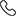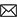Sales :9214233303 / Tech. Support Desk :9214233360/40 (9AM ~ 6PM)# How to Calculate JEE Mains Percentile ! Check Important Points Here

23 Jun, 2020, By Tanvi SharmaJEE Mains Percentile Score: The NTA conducts the JEE paper every year. The first and the foremost thing the students think about is How to calculate JEE Main Percentile score? The NTA declares the rank of the JEE main paper. The students are given two opportunities to appear for the JEE main paper. The better score of the student is considered to check the percentile.

Once the student is done with the JEE main he/she is very eager to know the percentile, so here we have the entire process for you how you can calculate percentile scores of jee main. But before that, you need to understand the accurate meaning of the JEE percentile and what’s the need to know the percentile score?

Read Also: JEE Main Difficulty Level 2023: Level Up Your Strategies For JEE 2023

## How to Calculate JEE Mains Percentile Score?

Calculate JEE Mains Percentile-what is the meaning of the JEE main percentile score?

As you must be knowing that the maximum marks of the JEE main paper are 300. Now let us suppose that a student got 260 out of 300, then as the highest marks scored by any student is 260; 260 will be considered as the percentile score. So how to calculate JEE Main 2023 rank/NTA Percentile score from the highest marks.

To derive you’re JEE Main NTA score 2023 consider the below-given formula:

Percentile Score of a Candidate = 100 x (Number of candidates who secured a raw score (or actual score) EQUAL TO OR LESS than the candidate) / (Total number of candidates who appeared in that session).

Apply the formula and you will have your NTA score or what you call as jee main nta percentile score 2023. This will help you know how you performed in your paper as compared to other candidates.

Also Read: All About Study of PCM for the JEE - The right guide for your study### Important points to know about JEE Mains Percentile Score

• Percentage and percentile score are two different things

• The merit list is released on the basis of the percentile scores of the students and not on the basis of the raw marks scored

• The percentile you have scored in your shift plays an important role

• Formula to know your percentile: (No. of students in your shift with a score greater than or equal to your score) / (total number of students in your shift)

• the tie-breaker for the 8 students with percentile 90 in Shift 1 and percentile 90 in Shift 2 and so on will be on the basis of

• math’s percentile followed by

• physics’ percentile followed by

• chemistry’s percentile followed by

• age (older twins)

Also Read: Best learning resources for JEE 2023 preparation

You have a second option with you in case your percentile score was not good in the January session. You can multiply your efforts for the second session and can improve your jee mains percentile score and as said the percentile score that is higher will be taken into consideration.

Keep following EtoosIndia JEE Articles for the latest updates.